# RS Aggarwal Solutions for Class 6 Maths Chapter 10 Ratio, Proportion and Unitary Method

RS Aggarwal Solutions for Class 6 Chapter 10 Ratio, Proportion and Unitary Method are given here. Students who practice RS Aggarwal Solutions regularly will excel with good marks and gain confidence in Mathematics. By going through RS Aggarwal textbook questions and solving them will help the students to clearly understand the topics related to this chapter.

Students are advised to go through RS Aggarwal Solutions for Class 6 Maths Chapter 10 which have been solved by experts in Mathematics and are available in PDF format. Download PDF of Class 6 Chapter 10 in their respective links.

## Download PDF of RS Aggarwal Solutions for Class 6 Maths Chapter 10 Ratio, Proportion and Unitary Method.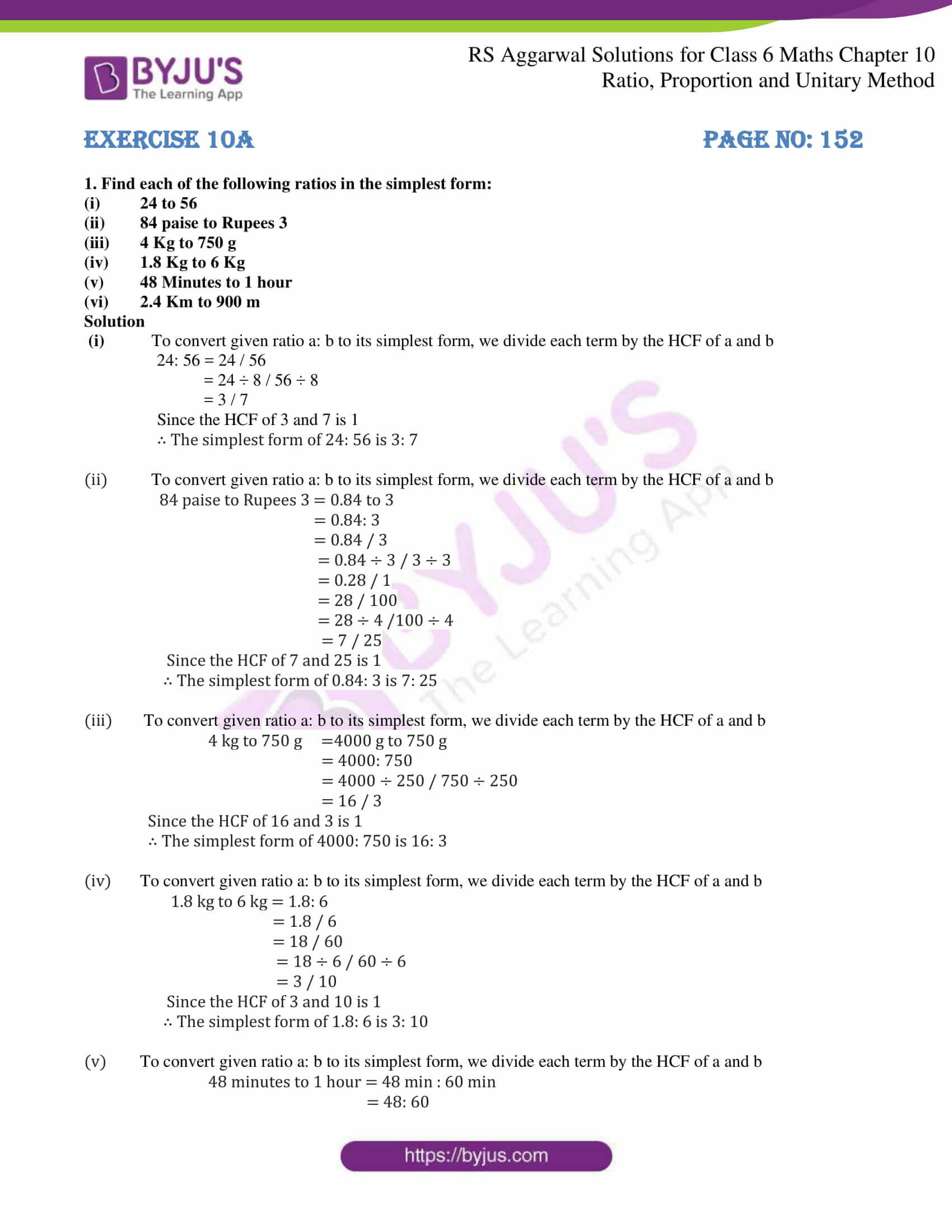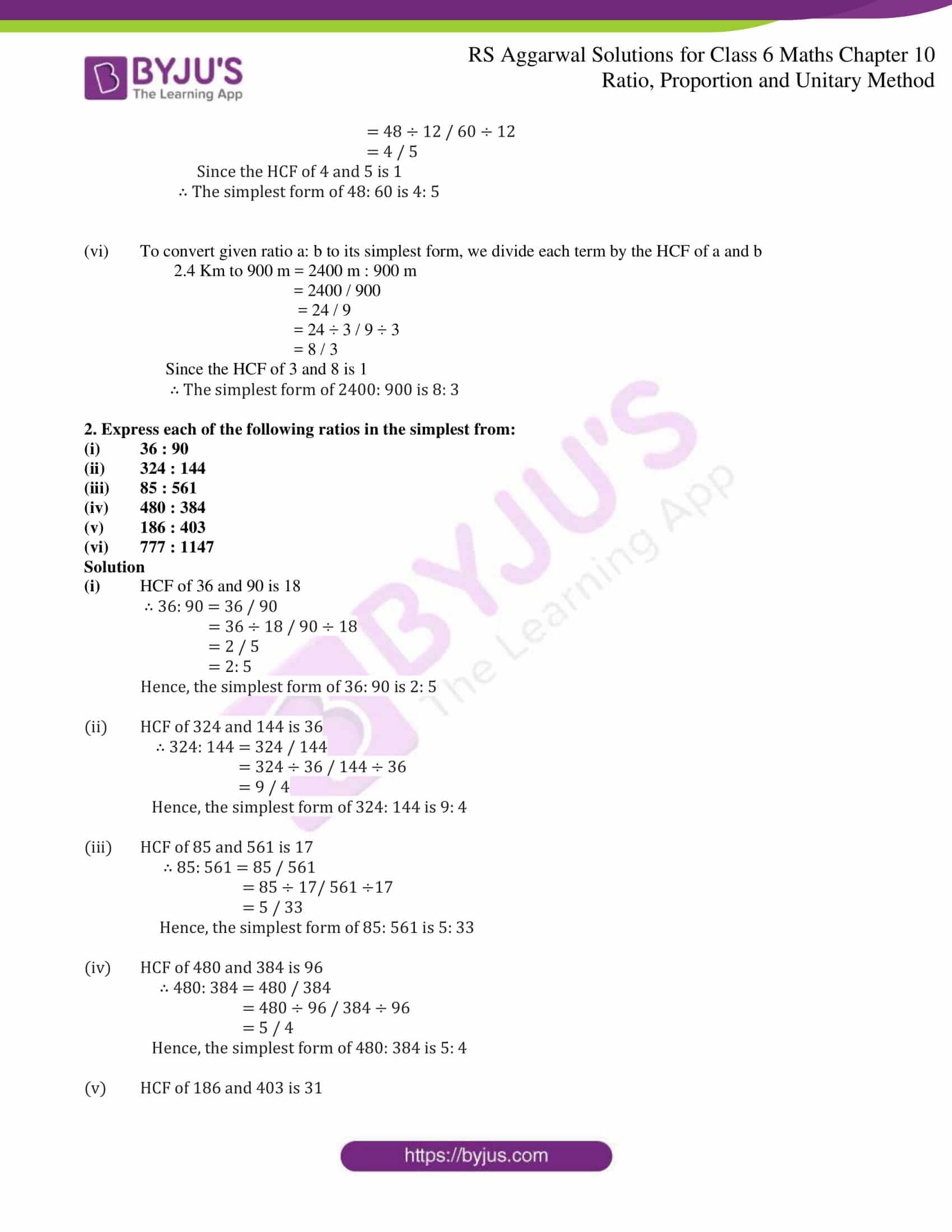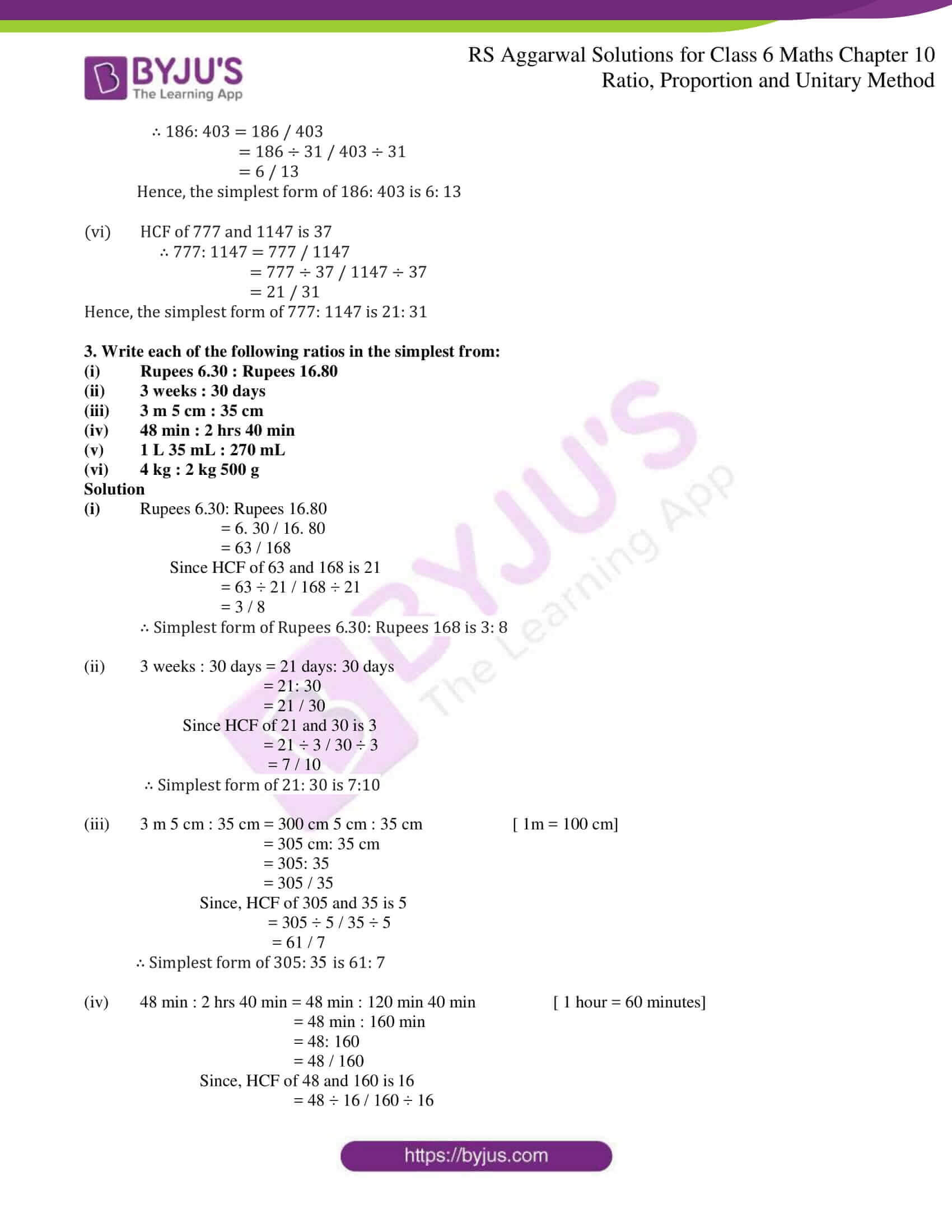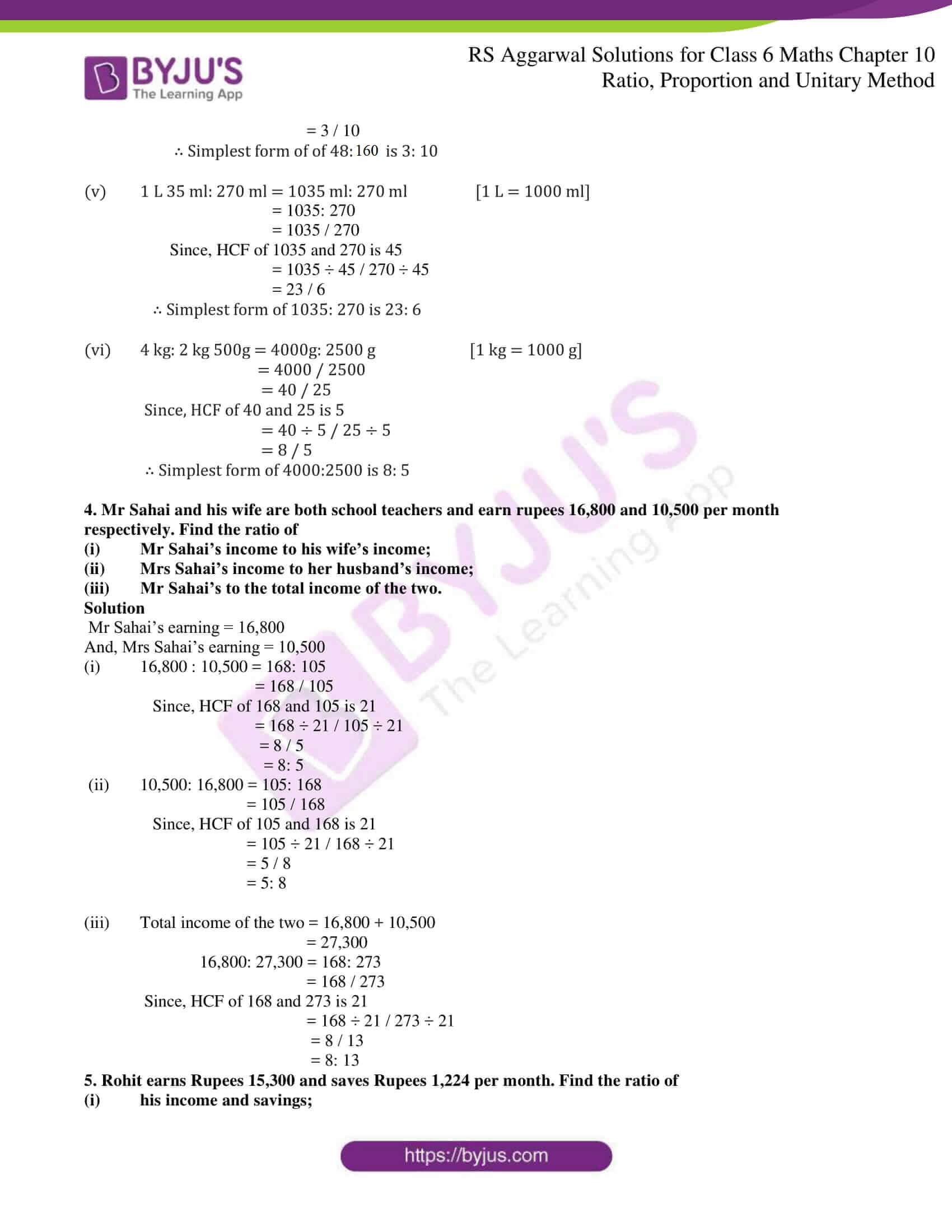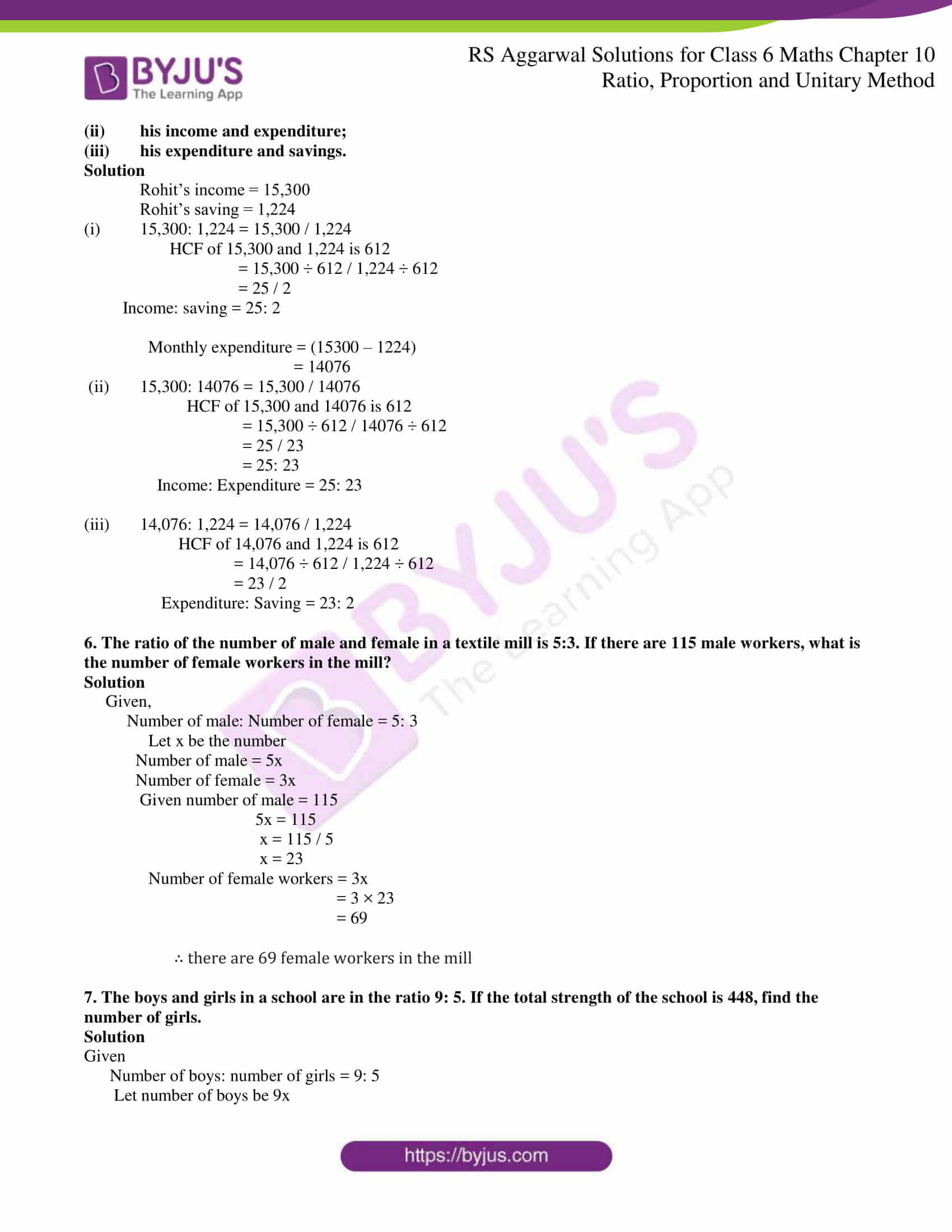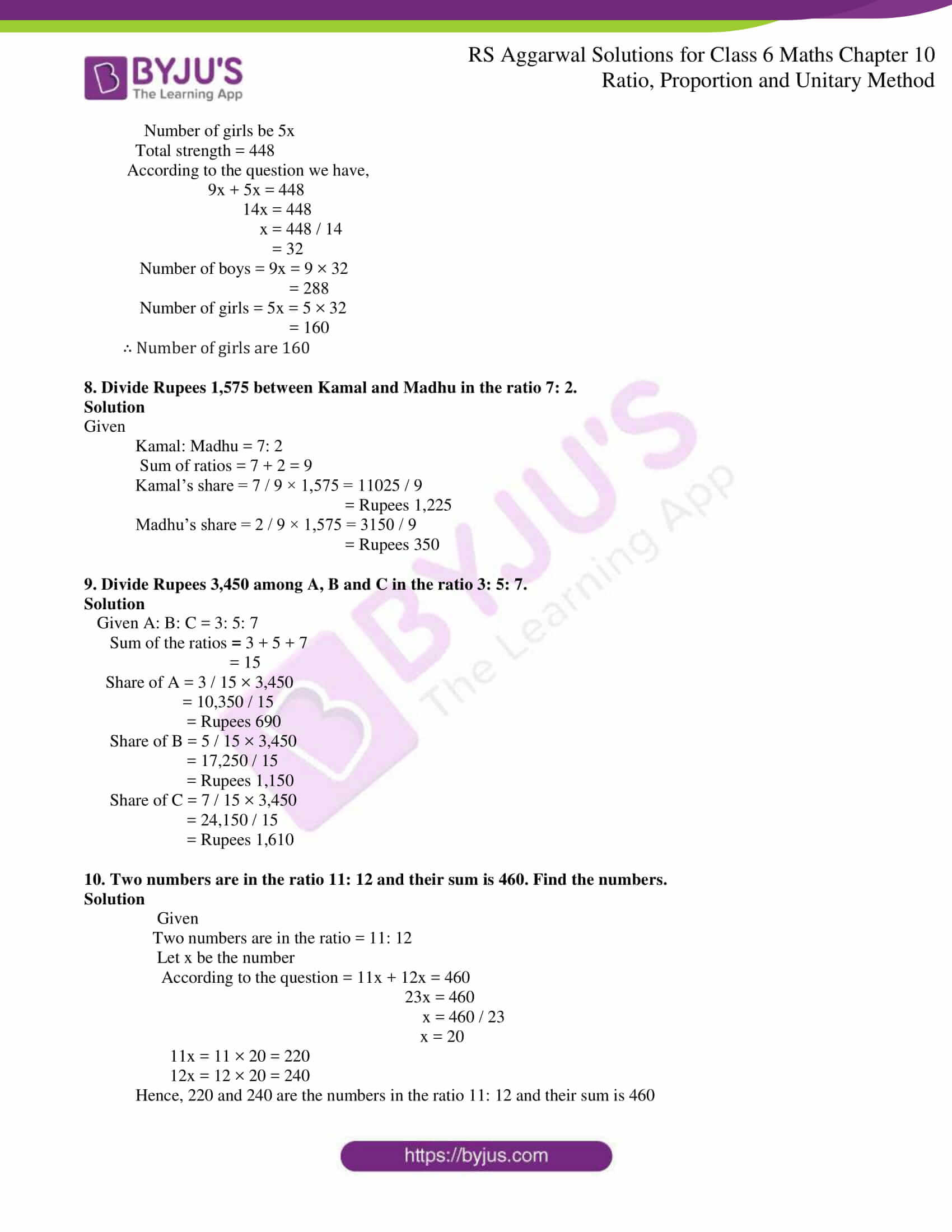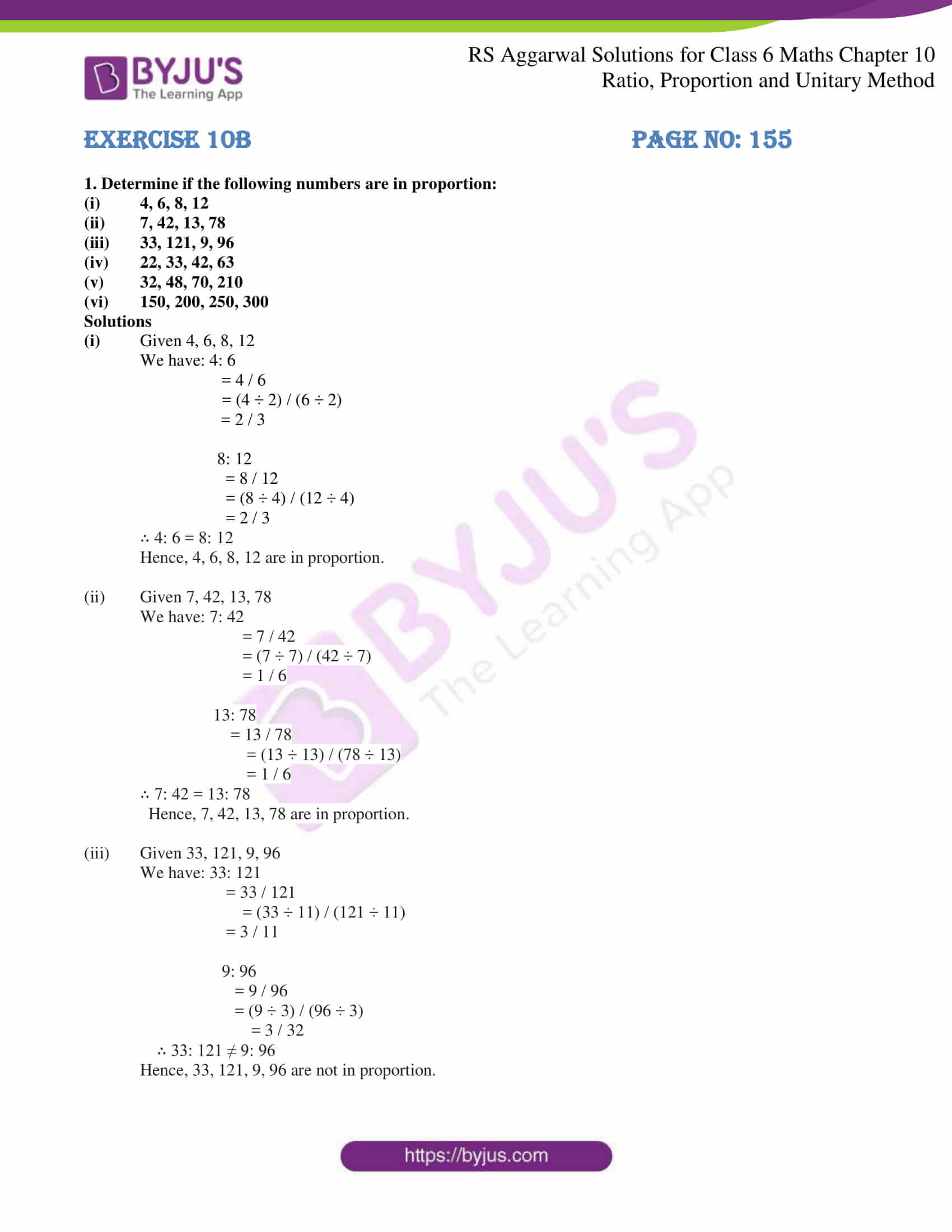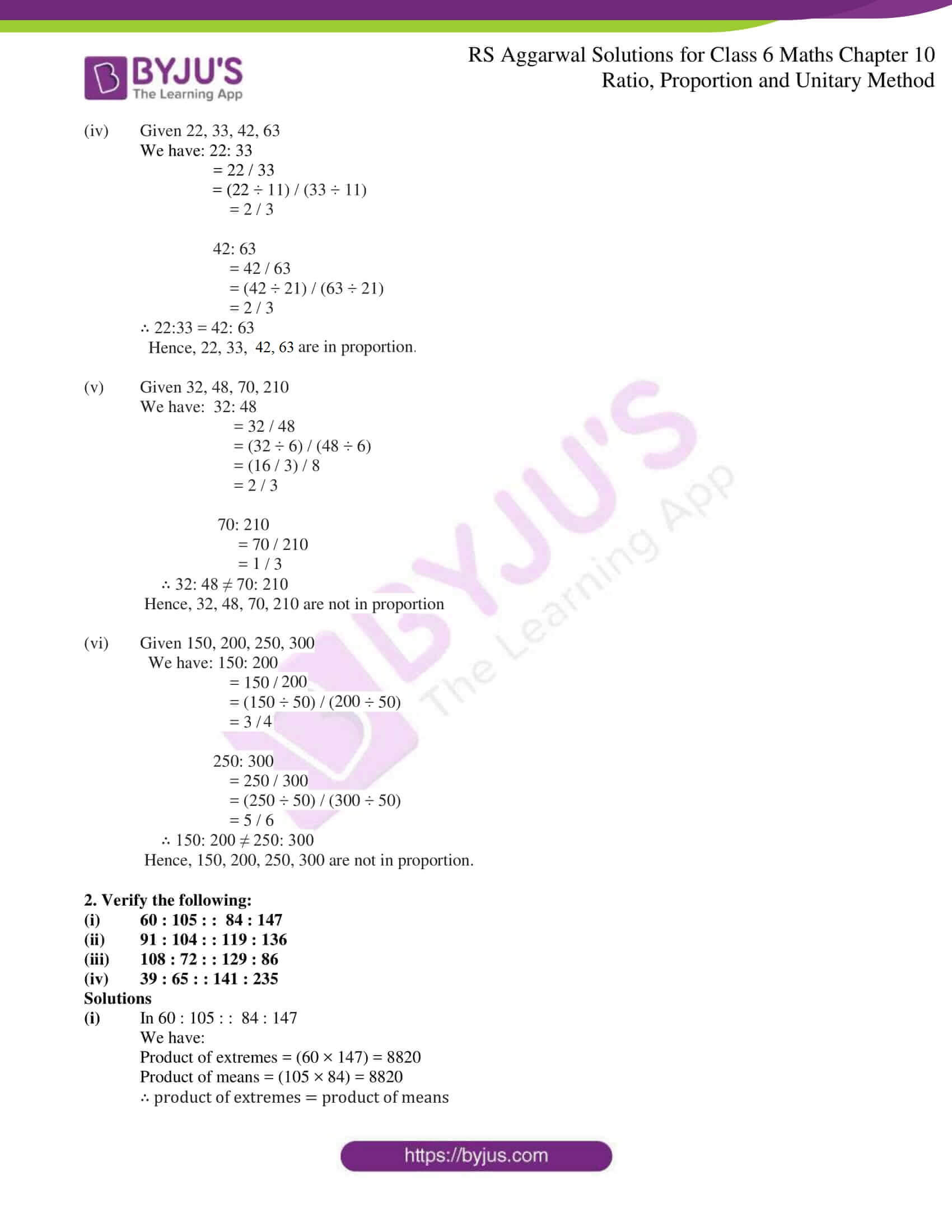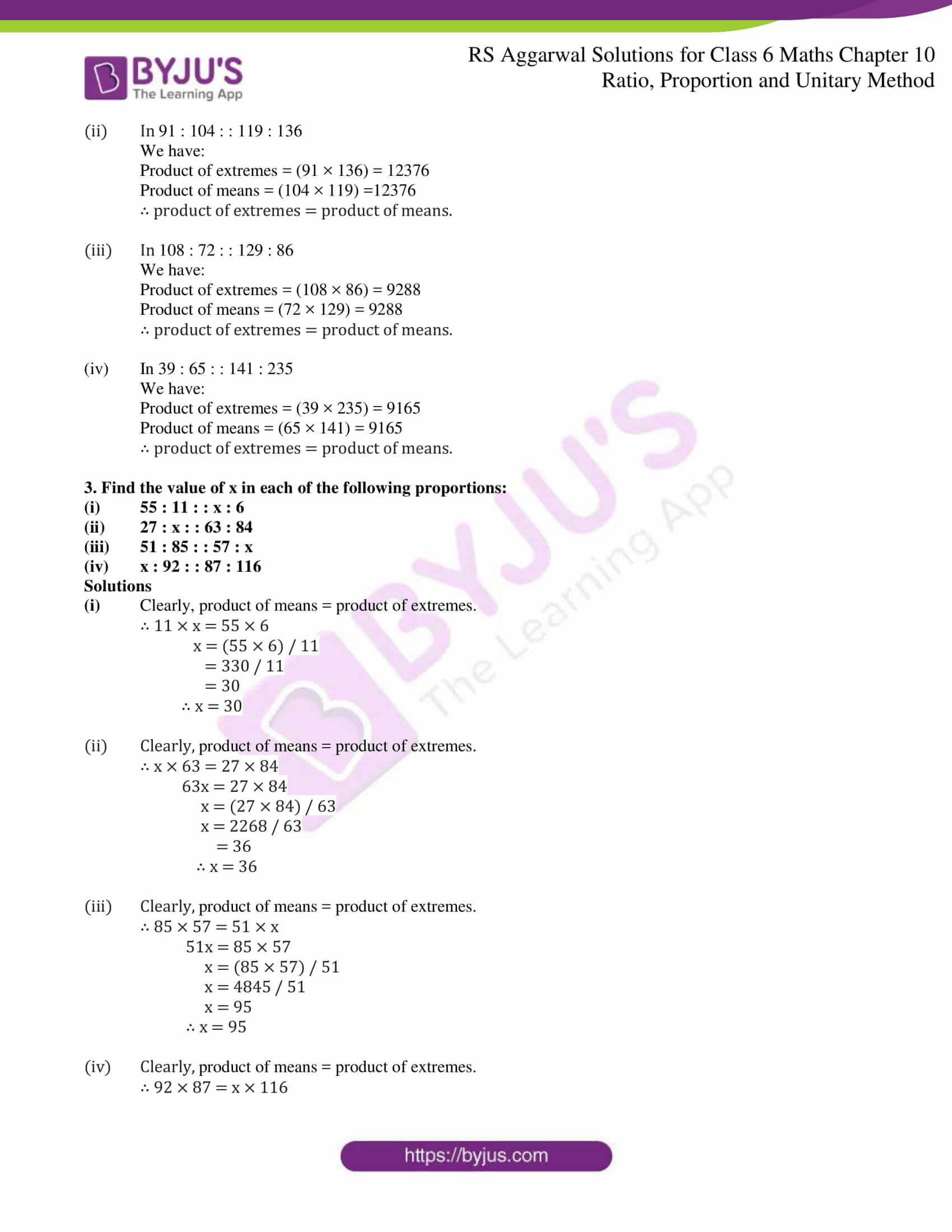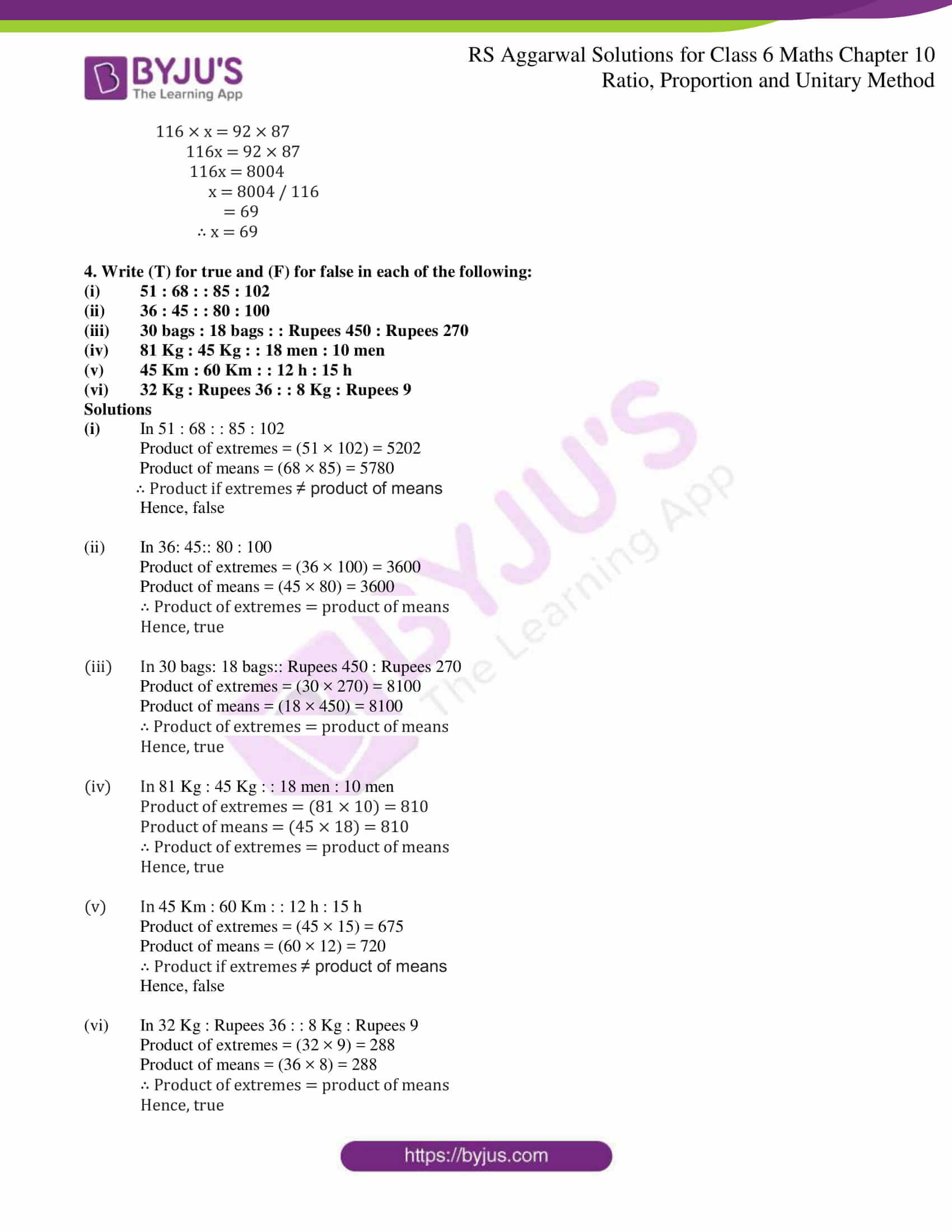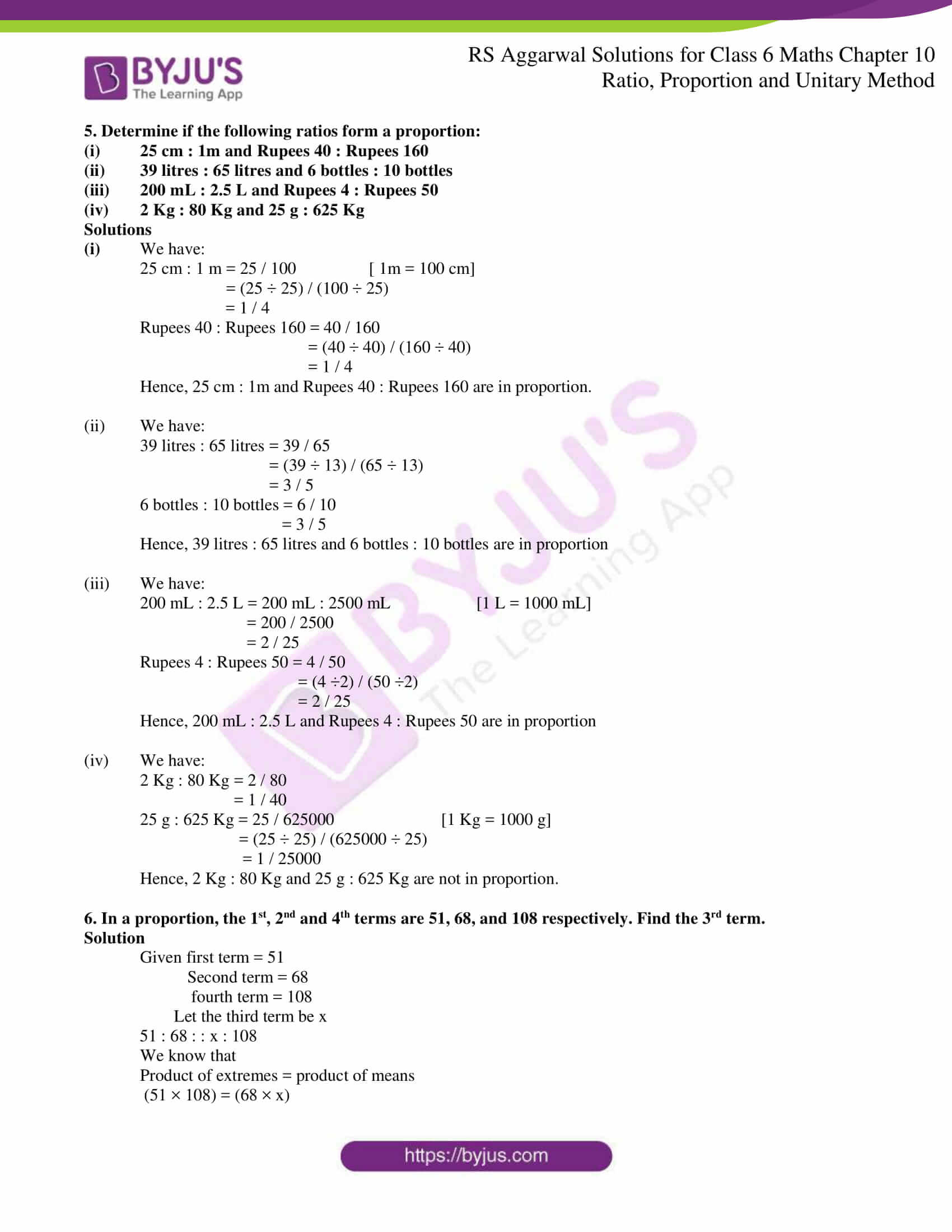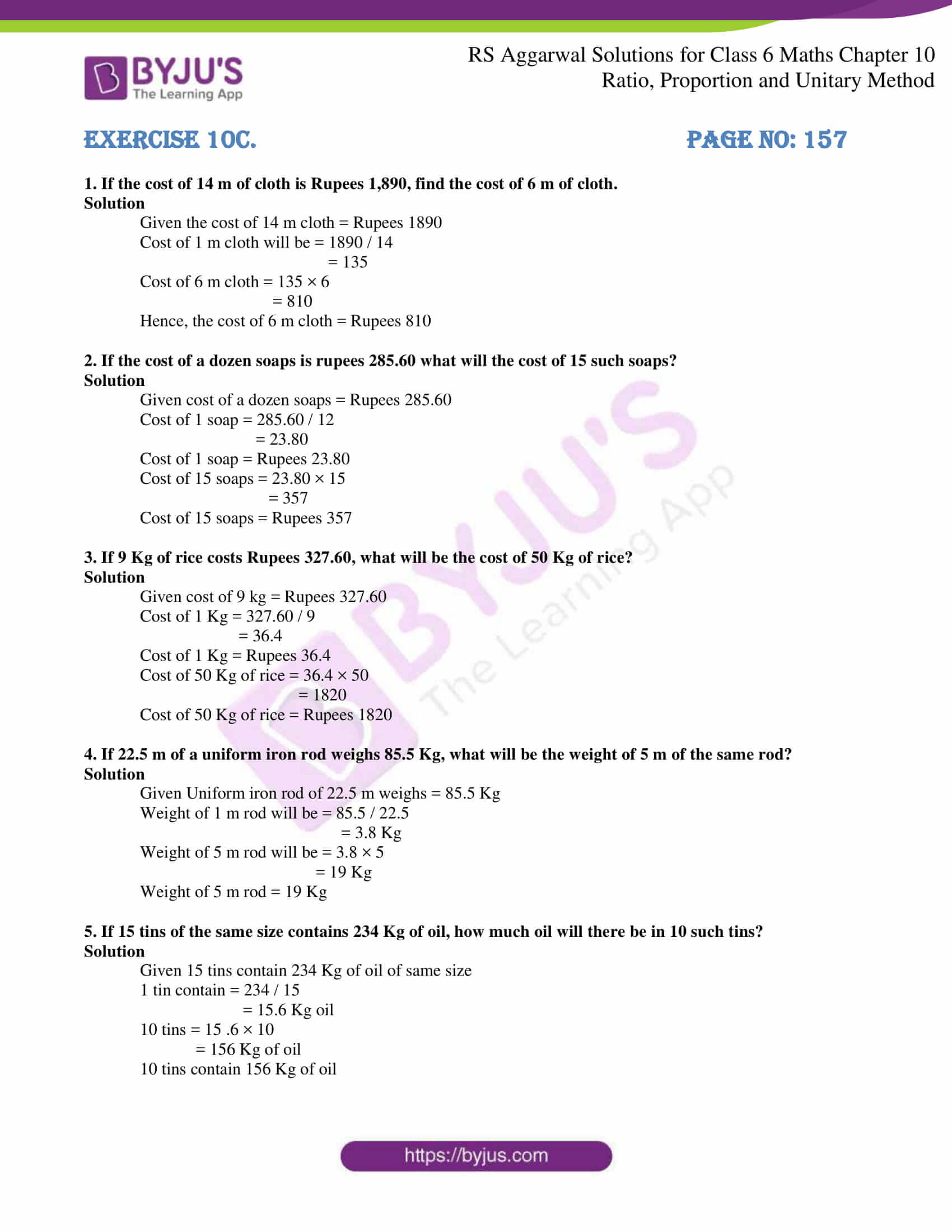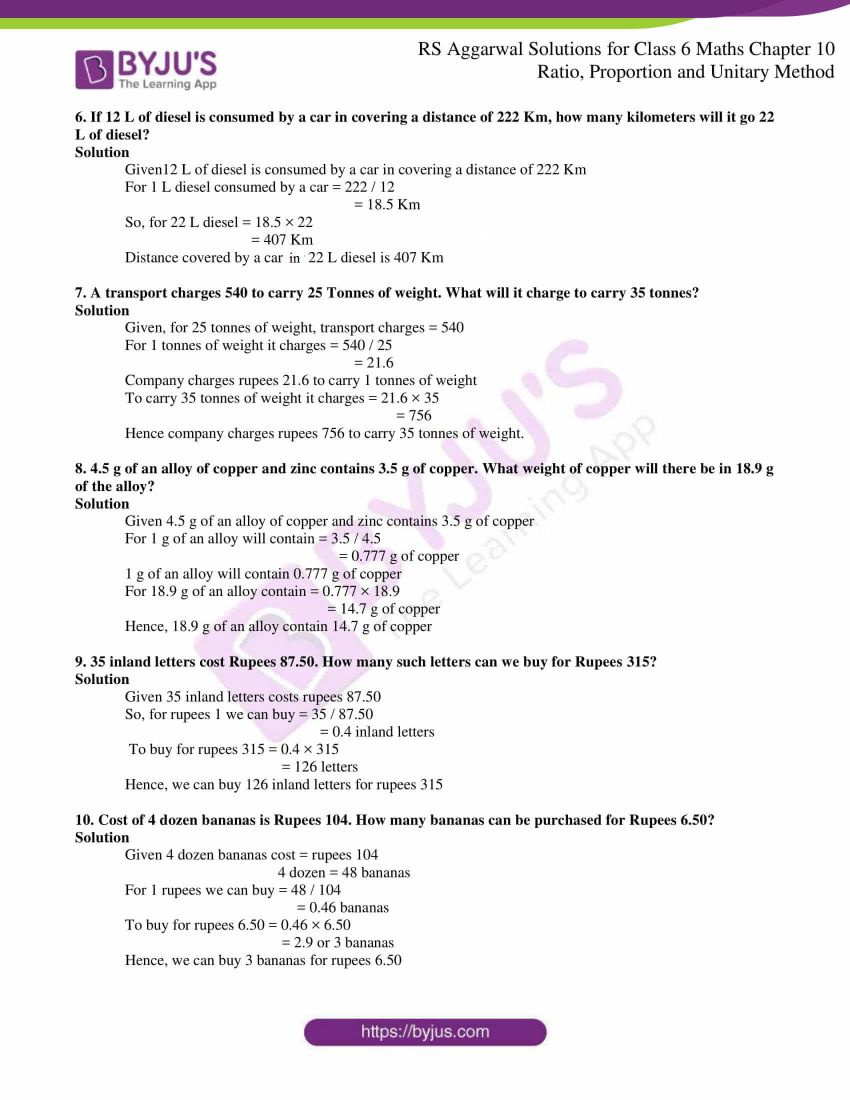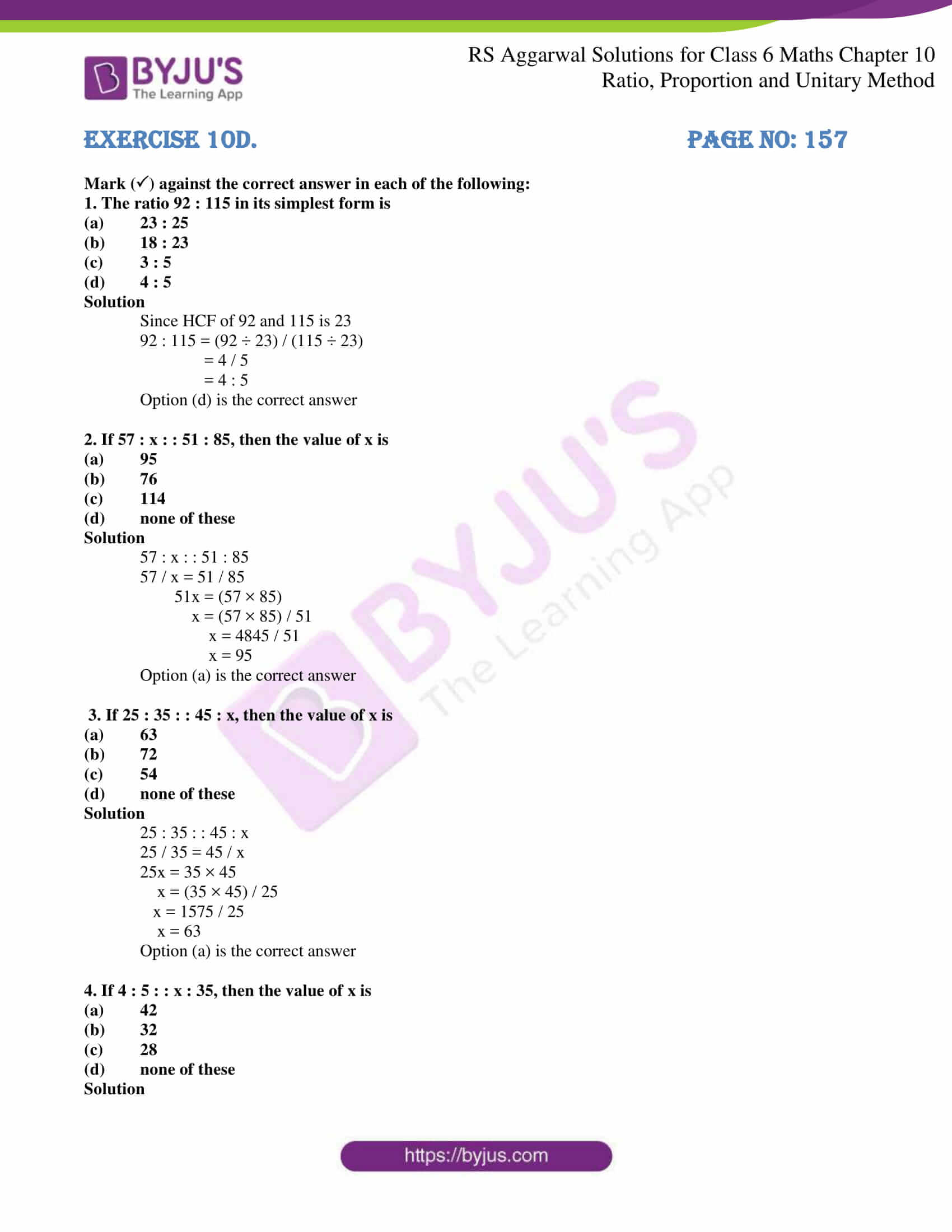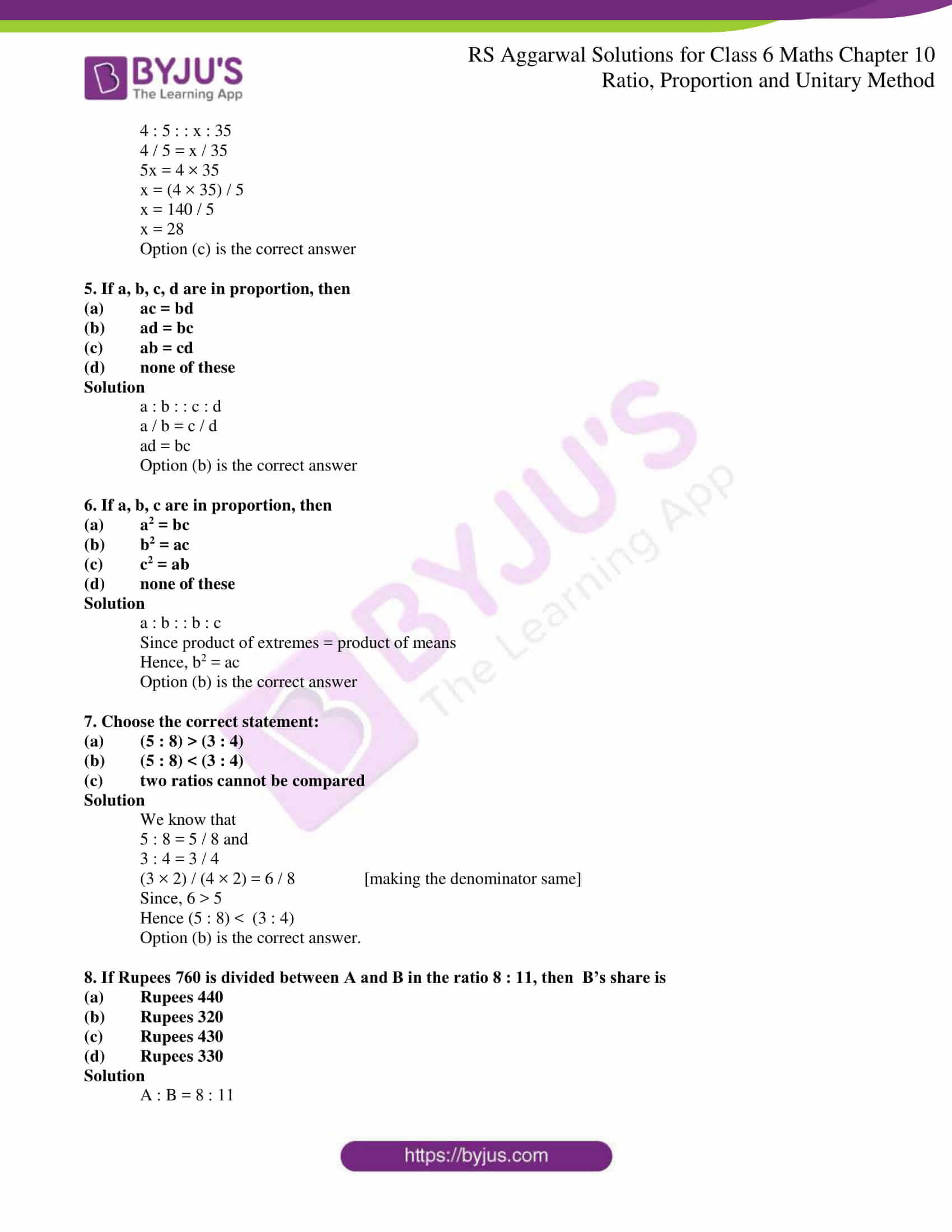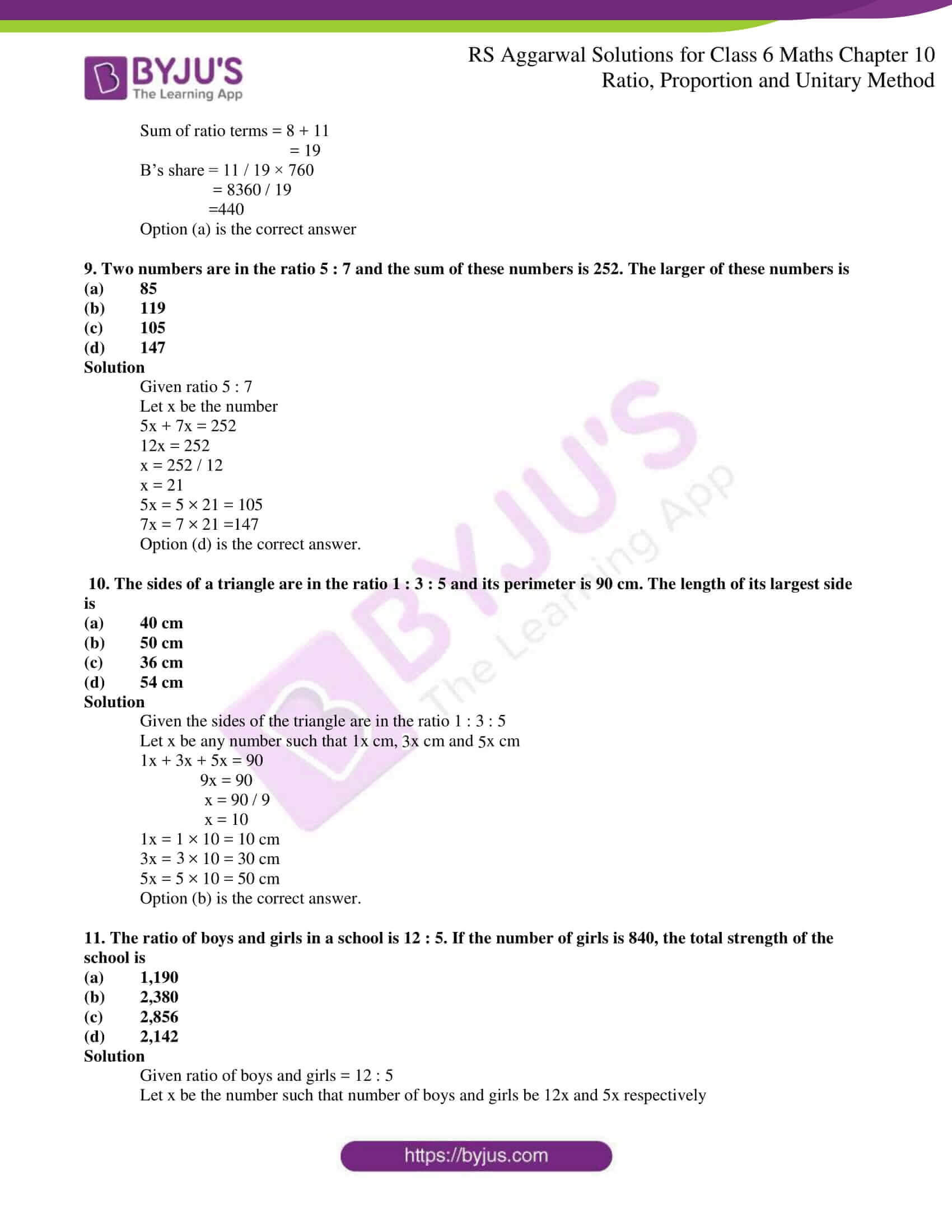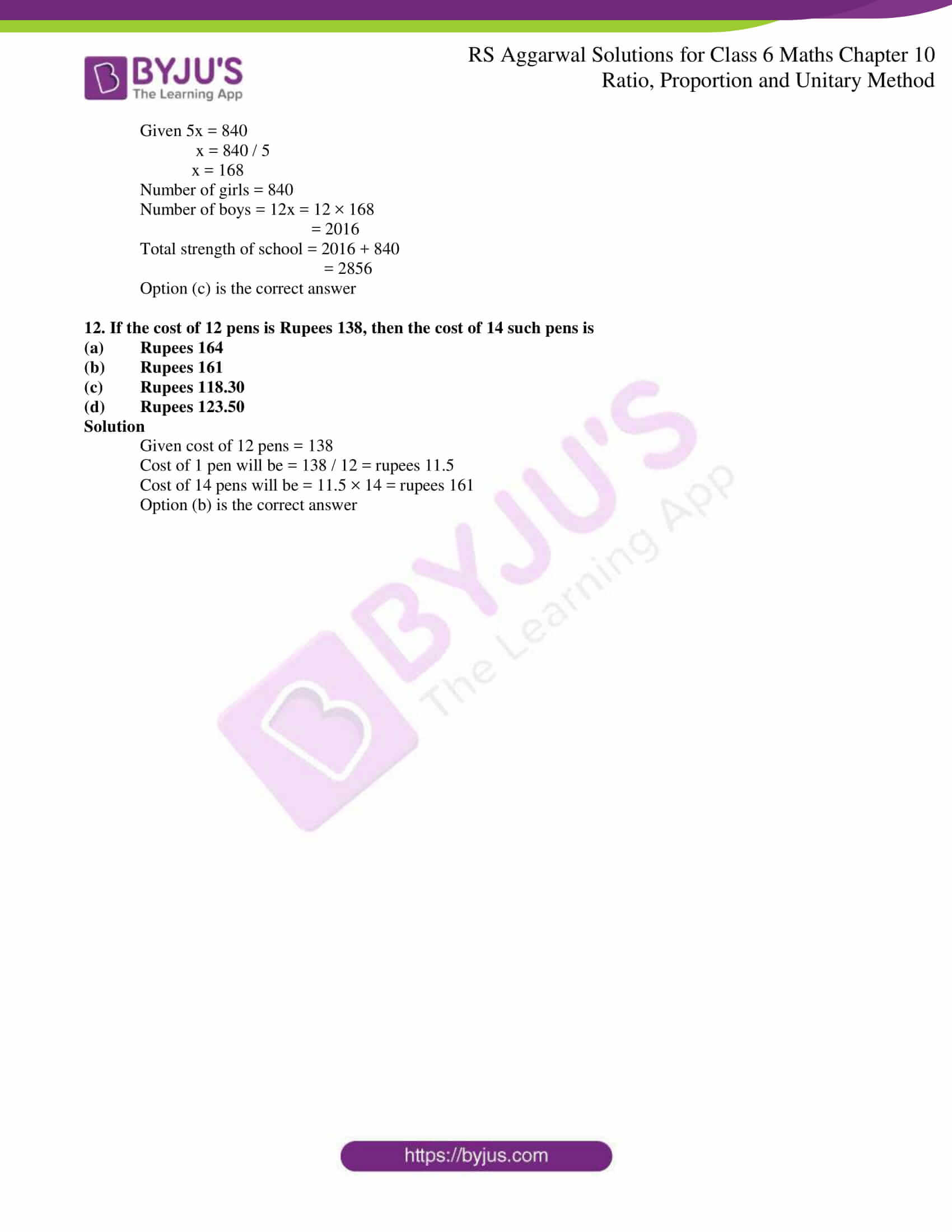### Access answers of RS Aggarwal Solutions for Class 6 Maths Chapter 10 – Ratio, Proportion and Unitary Method

Exercise 10A PAGE NO: 152

1. Find each of the following ratios in the simplest form:

(i) 24 to 56

(ii) 84 paise to Rupees 3

(iii) 4 Kg to 750 g

(iv) 1.8 Kg to 6 Kg

(v) 48 Minutes to 1 hour

(vi) 2.4 Km to 900 m

Solution

(i) To convert given ratio a: b to its simplest form, we divide each term by the HCF of a and b

24: 56 = 24 / 56

= 24 ÷ 8 / 56 ÷ 8

= 3 / 7

Since the HCF of 3 and 7 is 1

∴ The simplest form of 24: 56 is 3: 7

(ii) To convert given ratio a: b to its simplest form, we divide each term by the HCF of a and b

84 paise to Rupees 3 = 0.84 to 3

= 0.84: 3

= 0.84 / 3

= 0.84 ÷ 3 / 3 ÷ 3

= 0.28 / 1

= 28 / 100

= 28 ÷ 4 /100 ÷ 4

= 7 / 25

Since the HCF of 7 and 25 is 1

∴ The simplest form of 0.84: 3 is 7: 25

(iii) To convert given ratio a: b to its simplest form, we divide each term by the HCF of a and b

4 kg to 750 g =4000 g to 750 g

= 4000: 750

= 4000 ÷ 250 / 750 ÷ 250

= 16 / 3

Since the HCF of 16 and 3 is 1

∴ The simplest form of 4000: 750 is 16: 3

(iv) To convert given ratio a: b to its simplest form, we divide each term by the HCF of a and b

1.8 kg to 6 kg = 1.8: 6

= 1.8 / 6

= 18 / 60

= 18 ÷ 6 / 60 ÷ 6

= 3 / 10

Since the HCF of 3 and 10 is 1

∴ The simplest form of 1.8: 6 is 3: 10

(v) To convert given ratio a: b to its simplest form, we divide each term by the HCF of a and b

48 minutes to 1 hour = 48 min : 60 min

= 48: 60

= 48 ÷ 12 / 60 ÷ 12

= 4 / 5

Since the HCF of 4 and 5 is 1

∴ The simplest form of 48: 60 is 4: 5

(vi) To convert given ratio a: b to its simplest form, we divide each term by the HCF of a and b

2.4 Km to 900 m = 2400 m : 900 m

= 2400 / 900

= 24 / 9

= 24 ÷ 3 / 9 ÷ 3

= 8 / 3

Since the HCF of 3 and 8 is 1

∴ The simplest form of 2400: 900 is 8: 3

2. Express each of the following ratios in the simplest from:

(i) 36 : 90

(ii) 324 : 144

(iii) 85 : 561

(iv) 480 : 384

(v) 186 : 403

(vi) 777 : 1147

Solution

(i) HCF of 36 and 90 is 18

∴ 36: 90 = 36 / 90

= 36 ÷ 18 / 90 ÷ 18

= 2 / 5

= 2: 5

Hence, the simplest form of 36: 90 is 2: 5

(ii) HCF of 324 and 144 is 36

∴ 324: 144 = 324 / 144

= 324 ÷ 36 / 144 ÷ 36

= 9 / 4

Hence, the simplest form of 324: 144 is 9: 4

(iii) HCF of 85 and 561 is 17

∴ 85: 561 = 85 / 561

= 85 ÷ 17/ 561 ÷17

= 5 / 33

Hence, the simplest form of 85: 561 is 5: 33

(iv) HCF of 480 and 384 is 96

∴ 480: 384 = 480 / 384

= 480 ÷ 96 / 384 ÷ 96

= 5 / 4

Hence, the simplest form of 480: 384 is 5: 4

(v) HCF of 186 and 403 is 31

∴ 186: 403 = 186 / 403

= 186 ÷ 31 / 403 ÷ 31

= 6 / 13

Hence, the simplest form of 186: 403 is 6: 13

(vi) HCF of 777 and 1147 is 37

∴ 777: 1147 = 777 / 1147

= 777 ÷ 37 / 1147 ÷ 37

= 21 / 31

Hence, the simplest form of 777: 1147 is 21: 31

3. Write each of the following ratios in the simplest from:

(i) Rupees 6.30 : Rupees 16.80

(ii) 3 weeks : 30 days

(iii) 3 m 5 cm : 35 cm

(iv) 48 min : 2 hrs 40 min

(v) 1 L 35 mL : 270 mL

(vi) 4 kg : 2 kg 500 g

Solution

(i) Rupees 6.30: Rupees 16.80

= 6. 30 / 16. 80

= 63 / 168

Since HCF of 63 and 168 is 21

= 63 ÷ 21 / 168 ÷ 21

= 3 / 8

∴ Simplest form of Rupees 6.30: Rupees 168 is 3: 8

(ii) 3 weeks : 30 days = 21 days: 30 days

= 21: 30

= 21 / 30

Since HCF of 21 and 30 is 3

= 21 ÷ 3 / 30 ÷ 3

= 7 / 10

∴ Simplest form of 21: 30 is 7:10

(iii) 3 m 5 cm : 35 cm = 300 cm 5 cm : 35 cm [ 1m = 100 cm]

= 305 cm: 35 cm

= 305: 35

= 305 / 35

Since, HCF of 305 and 35 is 5

= 305 ÷ 5 / 35 ÷ 5

= 61 / 7

∴ Simplest form of 305: 35 is 61: 7

(iv) 48 min : 2 hrs 40 min = 48 min : 120 min 40 min [ 1 hour = 60 minutes]

= 48 min : 160 min

= 48: 160

= 48 / 160

Since, HCF of 48 and 160 is 16

= 48 ÷ 16 / 160 ÷ 16

= 3 / 10

∴ Simplest form of of 48:160 is 3: 10

(v) 1 L 35 ml: 270 ml = 1035 ml: 270 ml [1 L = 1000 ml]

= 1035: 270

= 1035 / 270

Since, HCF of 1035 and 270 is 45

= 1035 ÷ 45 / 270 ÷ 45

= 23 / 6

∴ Simplest form of 1035: 270 is 23: 6

(vi) 4 kg: 2 kg 500g = 4000g: 2500 g [1 kg = 1000 g]

= 4000 / 2500

= 40 / 25

Since, HCF of 40 and 25 is 5

= 40 ÷ 5 / 25 ÷ 5

= 8 / 5

∴ Simplest form of 4000:2500 is 8: 5

4. Mr Sahai and his wife are both school teachers and earn rupees 16,800 and 10,500 per month respectively. Find the ratio of

(i) Mr Sahai’s income to his wife’s income;

(ii) Mrs Sahai’s income to her husband’s income;

(iii) Mr Sahai’s to the total income of the two.

Solution

Mr Sahai’s earning = 16,800

And, Mrs Sahai’s earning = 10,500

(i) 16,800 : 10,500 = 168: 105

= 168 / 105

Since, HCF of 168 and 105 is 21

= 168 ÷ 21 / 105 ÷ 21

= 8 / 5

= 8: 5

(ii) 10,500: 16,800 = 105: 168

= 105 / 168

Since, HCF of 105 and 168 is 21

= 105 ÷ 21 / 168 ÷ 21

= 5 / 8

= 5: 8

(iii) Total income of the two = 16,800 + 10,500

= 27,300

16,800: 27,300 = 168: 273

= 168 / 273

Since, HCF of 168 and 273 is 21

= 168 ÷ 21 / 273 ÷ 21

= 8 / 13

= 8: 13

5. Rohit earns Rupees 15,300 and saves Rupees 1,224 per month. Find the ratio of

(i) his income and savings;

(ii) his income and expenditure;

(iii) his expenditure and savings.

Solution

Rohit’s income = 15,300

Rohit’s saving = 1,224

(i) 15,300: 1,224 = 15,300 / 1,224

HCF of 15,300 and 1,224 is 612

= 15,300 ÷ 612 / 1,224 ÷ 612

= 25 / 2

Income: saving = 25: 2

Monthly expenditure = (15300 – 1224)

= 14076

(ii) 15,300: 14076 = 15,300 / 14076

HCF of 15,300 and 14076 is 612

= 15,300 ÷ 612 / 14076 ÷ 612

= 25 / 23

= 25: 23

Income: Expenditure = 25: 23

(iii) 14,076: 1,224 = 14,076 / 1,224

HCF of 14,076 and 1,224 is 612

= 14,076 ÷ 612 / 1,224 ÷ 612

= 23 / 2

Expenditure: Saving = 23: 2

6. The ratio of the number of male and female in a textile mill is 5:3. If there are 115 male workers, what is the number of female workers in the mill?

Solution

Given,

Number of male: Number of female = 5: 3

Let x be the number

Number of male = 5x

Number of female = 3x

Given number of male = 115

5x = 115

x = 115 / 5

x = 23

Number of female workers = 3x

= 3 × 23

= 69

∴ there are 69 female workers in the mill

7. The boys and girls in a school are in the ratio 9: 5. If the total strength of the school is 448, find the number of girls.

Solution

Given

Number of boys: number of girls = 9: 5

Let number of boys be 9x

Number of girls be 5x

Total strength = 448

According to the question we have,

9x + 5x = 448

14x = 448

x = 448 / 14

= 32

Number of boys = 9x = 9 × 32

= 288

Number of girls = 5x = 5 × 32

= 160

∴ Number of girls are 160

8. Divide Rupees 1,575 between Kamal and Madhu in the ratio 7: 2.

Solution

Given

Sum of ratios = 7 + 2 = 9

Kamal’s share = 7 / 9 × 1,575 = 11025 / 9

= Rupees 1,225

Madhu’s share = 2 / 9 × 1,575 = 3150 / 9

= Rupees 350

9. Divide Rupees 3,450 among A, B and C in the ratio 3: 5: 7.

Solution

Given A: B: C = 3: 5: 7

Sum of the ratios = 3 + 5 + 7

= 15

Share of A = 3 / 15 × 3,450

= 10,350 / 15

= Rupees 690

Share of B = 5 / 15 × 3,450

= 17,250 / 15

= Rupees 1,150

Share of C = 7 / 15 × 3,450

= 24,150 / 15

= Rupees 1,610

10. Two numbers are in the ratio 11: 12 and their sum is 460. Find the numbers.

Solution

Given

Two numbers are in the ratio = 11: 12

Let x be the number

According to the question = 11x + 12x = 460

23x = 460

x = 460 / 23

x = 20

11x = 11 × 20 = 220

12x = 12 × 20 = 240

Hence, 220 and 240 are the numbers in the ratio 11: 12 and their sum is 460

Exercise 10B PAGE NO: 155

1. Determine if the following numbers are in proportion:

(i) 4, 6, 8, 12

(ii) 7, 42, 13, 78

(iii) 33, 121, 9, 96

(iv) 22, 33, 42, 63

(v) 32, 48, 70, 210

(vi) 150, 200, 250, 300

Solutions

(i) Given 4, 6, 8, 12

We have: 4: 6

= 4 / 6

= (4 ÷ 2) / (6 ÷ 2)

= 2 / 3

8: 12

= 8 / 12

= (8 ÷ 4) / (12 ÷ 4)

= 2 / 3

∴ 4: 6 = 8: 12

Hence, 4, 6, 8, 12 are in proportion.

(ii) Given 7, 42, 13, 78

We have: 7: 42

= 7 / 42

= (7 ÷ 7) / (42 ÷ 7)

= 1 / 6

13: 78

= 13 / 78

= (13 ÷ 13) / (78 ÷ 13)

= 1 / 6

∴ 7: 42 = 13: 78

Hence, 7, 42, 13, 78 are in proportion.

(iii) Given 33, 121, 9, 96

We have: 33: 121

= 33 / 121

= (33 ÷ 11) / (121 ÷ 11)

= 3 / 11

9: 96

= 9 / 96

= (9 ÷ 3) / (96 ÷ 3)

= 3 / 32

∴ 33: 121 ≠ 9: 96

Hence, 33, 121, 9, 96 are not in proportion.

(iv) Given 22, 33, 42, 63

We have: 22: 33

= 22 / 33

= (22 ÷ 11) / (33 ÷ 11)

= 2 / 3

42: 63

= 42 / 63

= (42 ÷ 21) / (63 ÷ 21)

= 2 / 3

∴ 22:33 = 42: 63

Hence, 22, 33, 42, 63 are in proportion.

(v) Given 32, 48, 70, 210

We have: 32: 48

= 32 / 48

= (32 ÷ 6) / (48 ÷ 6)

= (16 / 3) / 8

= 2 / 3

70: 210

= 70 / 210

= 1 / 3

∴ 32: 48 ≠ 70: 210

Hence, 32, 48, 70, 210 are not in proportion

(vi) Given 150, 200, 250, 300

We have: 150: 200

= 150 / 200

= (150 ÷ 50) / (200 ÷ 50)

= 3 / 4

250: 300

= 250 / 300

= (250 ÷ 50) / (300 ÷ 50)

= 5 / 6

∴ 150: 200 ≠ 250: 300

Hence, 150, 200, 250, 300 are not in proportion.

2. Verify the following:

(i) 60 : 105 : : 84 : 147

(ii) 91 : 104 : : 119 : 136

(iii) 108 : 72 : : 129 : 86

(iv) 39 : 65 : : 141 : 235

Solutions

(i) In 60 : 105 : : 84 : 147

We have:

Product of extremes = (60 × 147) = 8820

Product of means = (105 × 84) = 8820

∴ product of extremes = product of means

(ii) In 91 : 104 : : 119 : 136

We have:

Product of extremes = (91 × 136) = 12376

Product of means = (104 × 119) =12376

∴ product of extremes = product of means.

(iii) In 108 : 72 : : 129 : 86

We have:

Product of extremes = (108 × 86) = 9288

Product of means = (72 × 129) = 9288

∴ product of extremes = product of means.

(iv) In 39 : 65 : : 141 : 235

We have:

Product of extremes = (39 × 235) = 9165

Product of means = (65 × 141) = 9165

∴ product of extremes = product of means.

3. Find the value of x in each of the following proportions:

(i) 55 : 11 : : x : 6

(ii) 27 : x : : 63 : 84

(iii) 51 : 85 : : 57 : x

(iv) x : 92 : : 87 : 116

Solutions

(i) Clearly, product of means = product of extremes.

∴ 11 × x = 55 × 6

x = (55 × 6) / 11

= 330 / 11

= 30

∴ x = 30

(ii) Clearly, product of means = product of extremes.

∴ x × 63 = 27 × 84

63x = 27 × 84

x = (27 × 84) / 63

x = 2268 / 63

= 36

∴ x = 36

(iii) Clearly, product of means = product of extremes.

∴ 85 × 57 = 51 × x

51x = 85 × 57

x = (85 × 57) / 51

x = 4845 / 51

x = 95

∴ x = 95

(iv) Clearly, product of means = product of extremes.

∴ 92 × 87 = x × 116

116 × x = 92 × 87

116x = 92 × 87

116x = 8004

x = 8004 / 116

= 69

∴ x = 69

4. Write (T) for true and (F) for false in each of the following:

(i) 51 : 68 : : 85 : 102

(ii) 36 : 45 : : 80 : 100

(iii) 30 bags : 18 bags : : Rupees 450 : Rupees 270

(iv) 81 Kg : 45 Kg : : 18 men : 10 men

(v) 45 Km : 60 Km : : 12 h : 15 h

(vi) 32 Kg : Rupees 36 : : 8 Kg : Rupees 9

Solutions

(i) In 51 : 68 : : 85 : 102

Product of extremes = (51 × 102) = 5202

Product of means = (68 × 85) = 5780

∴ Product if extremes ≠ product of means

Hence, false

(ii) In 36: 45:: 80 : 100

Product of extremes = (36 × 100) = 3600

Product of means = (45 × 80) = 3600

∴ Product of extremes = product of means

Hence, true

(iii) In 30 bags: 18 bags:: Rupees 450 : Rupees 270

Product of extremes = (30 × 270) = 8100

Product of means = (18 × 450) = 8100

∴ Product of extremes = product of means

Hence, true

(iv) In 81 Kg : 45 Kg : : 18 men : 10 men

Product of extremes = (81 × 10) = 810

Product of means = (45 × 18) = 810

∴ Product of extremes = product of means

Hence, true

(v) In 45 Km : 60 Km : : 12 h : 15 h

Product of extremes = (45 × 15) = 675

Product of means = (60 × 12) = 720

∴ Product if extremes ≠ product of means

Hence, false

(vi) In 32 Kg : Rupees 36 : : 8 Kg : Rupees 9

Product of extremes = (32 × 9) = 288

Product of means = (36 × 8) = 288

∴ Product of extremes = product of means

Hence, true

5. Determine if the following ratios form a proportion:

(i) 25 cm : 1m and Rupees 40 : Rupees 160

(ii) 39 litres : 65 litres and 6 bottles : 10 bottles

(iii) 200 mL : 2.5 L and Rupees 4 : Rupees 50

(iv) 2 Kg : 80 Kg and 25 g : 625 Kg

Solutions

(i) We have:

25 cm : 1 m = 25 / 100 [ 1m = 100 cm]

= (25 ÷ 25) / (100 ÷ 25)

= 1 / 4

Rupees 40 : Rupees 160 = 40 / 160

= (40 ÷ 40) / (160 ÷ 40)

= 1 / 4

Hence, 25 cm : 1m and Rupees 40 : Rupees 160 are in proportion.

(ii) We have:

39 litres : 65 litres = 39 / 65

= (39 ÷ 13) / (65 ÷ 13)

= 3 / 5

6 bottles : 10 bottles = 6 / 10

= 3 / 5

Hence, 39 litres : 65 litres and 6 bottles : 10 bottles are in proportion

(iii) We have:

200 mL : 2.5 L = 200 mL : 2500 mL [1 L = 1000 mL]

= 200 / 2500

= 2 / 25

Rupees 4 : Rupees 50 = 4 / 50

= (4 ÷2) / (50 ÷2)

= 2 / 25

Hence, 200 mL : 2.5 L and Rupees 4 : Rupees 50 are in proportion

(iv) We have:

2 Kg : 80 Kg = 2 / 80

= 1 / 40

25 g : 625 Kg = 25 / 625000 [1 Kg = 1000 g]

= (25 ÷ 25) / (625000 ÷ 25)

= 1 / 25000

Hence, 2 Kg : 80 Kg and 25 g : 625 Kg are not in proportion.

6. In a proportion, the 1st, 2nd and 4th terms are 51, 68, and 108 respectively. Find the 3rd term.

Solution

Given first term = 51

Second term = 68

fourth term = 108

Let the third term be x

51 : 68 : : x : 108

We know that

Product of extremes = product of means

(51 × 108) = (68 × x)

(68 × x) = (51 × 108)

68x = 5508

x = 5508 / 68 = 81

Exercise 10C. PAGE NO: 157

1. If the cost of 14 m of cloth is Rupees 1,890, find the cost of 6 m of cloth.

Solution

Given the cost of 14 m cloth = Rupees 1890

Cost of 1 m cloth will be = 1890 / 14

= 135

Cost of 6 m cloth = 135 × 6

= 810

Hence, the cost of 6 m cloth = Rupees 810

2. If the cost of a dozen soaps is rupees 285.60 what will the cost of 15 such soaps?

Solution

Given cost of a dozen soaps = Rupees 285.60

Cost of 1 soap = 285.60 / 12

= 23.80

Cost of 1 soap = Rupees 23.80

Cost of 15 soaps = 23.80 × 15

= 357

Cost of 15 soaps = Rupees 357

3. If 9 Kg of rice costs Rupees 327.60, what will be the cost of 50 Kg of rice?

Solution

Given cost of 9 kg = Rupees 327.60

Cost of 1 Kg = 327.60 / 9

= 36.4

Cost of 1 Kg = Rupees 36.4

Cost of 50 Kg of rice = 36.4 × 50

= 1820

Cost of 50 Kg of rice = Rupees 1820

4. If 22.5 m of a uniform iron rod weighs 85.5 Kg, what will be the weight of 5 m of the same rod?

Solution

Given Uniform iron rod of 22.5 m weighs = 85.5 Kg

Weight of 1 m rod will be = 85.5 / 22.5

= 3.8 Kg

Weight of 5 m rod will be = 3.8 × 5

= 19 Kg

Weight of 5 m rod = 19 Kg

5. If 15 tins of the same size contains 234 Kg of oil, how much oil will there be in 10 such tins?

Solution

Given 15 tins contain 234 Kg of oil of same size

1 tin contain = 234 / 15

= 15.6 Kg oil

10 tins = 15 .6 × 10

= 156 Kg of oil

10 tins contain 156 Kg of oil

6. If 12 L of diesel is consumed by a car in covering a distance of 222 Km, how many kilometers will it go 22 L of diesel?

Solution

Given12 L of diesel is consumed by a car in covering a distance of 222 Km

For 1 L diesel consumed by a car = 222 / 12

= 18.5 Km

So, for 22 L diesel = 18.5 × 22

= 407 Km

Distance covered by a car in 22 L diesel is 407 Km

7. A transport charges 540 to carry 25 Tonnes of weight. What will it charge to carry 35 tonnes?

Solution

Given, for 25 tonnes of weight, transport charges = 540

For 1 tonnes of weight it charges = 540 / 25

= 21.6

Company charges rupees 21.6 to carry 1 tonnes of weight

To carry 35 tonnes of weight it charges = 21.6 × 35

= 756

Hence company charges rupees 756 to carry 35 tonnes of weight.

8. 4.5 g of an alloy of copper and zinc contains 3.5 g of copper. What weight of copper will there be in 18.9 g of the alloy?

Solution

Given 4.5 g of an alloy of copper and zinc contains 3.5 g of copper

For 1 g of an alloy will contain = 3.5 / 4.5

= 0.777 g of copper

1 g of an alloy will contain 0.777 g of copper

For 18.9 g of an alloy contain = 0.777 × 18.9

= 14.7 g of copper

Hence, 18.9 g of an alloy contain 14.7 g of copper

9. 35 inland letters cost Rupees 87.50. How many such letters can we buy for Rupees 315?

Solution

Given 35 inland letters costs rupees 87.50

So, for rupees 1 we can buy = 35 / 87.50

= 0.4 inland letters

To buy for rupees 315 = 0.4 × 315

= 126 letters

Hence, we can buy 126 inland letters for rupees 315

10. Cost of 4 dozen bananas is Rupees 104. How many bananas can be purchased for Rupees 6.50?

Solution

Given 4 dozen bananas cost = rupees 104

4 dozen = 48 bananas

For 1 rupees we can buy = 48 / 104

= 0.46 bananas

To buy for rupees 6.50 = 0.46 × 6.50

= 2.9 or 3 bananas

Hence, we can buy 3 bananas for rupees 6.50

Exercise 10D. PAGE NO: 157

Mark () against the correct answer in each of the following:

1. The ratio 92 : 115 in its simplest form is

(a) 23 : 25

(b) 18 : 23

(c) 3 : 5

(d) 4 : 5

Solution

Since HCF of 92 and 115 is 23

92 : 115 = (92 ÷ 23) / (115 ÷ 23)

= 4 / 5

= 4 : 5

Option (d) is the correct answer

2. If 57 : x : : 51 : 85, then the value of x is

(a) 95

(b) 76

(c) 114

(d) none of these

Solution

57 : x : : 51 : 85

57 / x = 51 / 85

51x = (57 × 85)

x = (57 × 85) / 51

x = 4845 / 51

x = 95

Option (a) is the correct answer

3. If 25 : 35 : : 45 : x, then the value of x is

(a) 63

(b) 72

(c) 54

(d) none of these

Solution

25 : 35 : : 45 : x

25 / 35 = 45 / x

25x = 35 × 45

x = (35 × 45) / 25

x = 1575 / 25

x = 63

Option (a) is the correct answer

4. If 4 : 5 : : x : 35, then the value of x is

(a) 42

(b) 32

(c) 28

(d) none of these

Solution

4 : 5 : : x : 35

4 / 5 = x / 35

5x = 4 × 35

x = (4 × 35) / 5

x = 140 / 5

x = 28

Option (c) is the correct answer

5. If a, b, c, d are in proportion, then

(a) ac = bd

(c) ab = cd

(d) none of these

Solution

a : b : : c : d

a / b = c / d

Option (b) is the correct answer

6. If a, b, c are in proportion, then

(a) a2 = bc

(b) b2 = ac

(c) c2 = ab

(d) none of these

Solution

a : b : : b : c

Since product of extremes = product of means

Hence, b2 = ac

Option (b) is the correct answer

7. Choose the correct statement:

(a) (5 : 8) > (3 : 4)

(b) (5 : 8) < (3 : 4)

(c) two ratios cannot be compared

Solution

We know that

5 : 8 = 5 / 8 and

3 : 4 = 3 / 4

(3 × 2) / (4 × 2) = 6 / 8 [making the denominator same]

Since, 6 > 5

Hence (5 : 8) < (3 : 4)

Option (b) is the correct answer.

8. If Rupees 760 is divided between A and B in the ratio 8 : 11, then B’s share is

(a) Rupees 440

(b) Rupees 320

(c) Rupees 430

(d) Rupees 330

Solution

A : B = 8 : 11

Sum of ratio terms = 8 + 11

= 19

B’s share = 11 / 19 × 760

= 8360 / 19

=440

Option (a) is the correct answer

9. Two numbers are in the ratio 5 : 7 and the sum of these numbers is 252. The larger of these numbers is

(a) 85

(b) 119

(c) 105

(d) 147

Solution

Given ratio 5 : 7

Let x be the number

5x + 7x = 252

12x = 252

x = 252 / 12

x = 21

5x = 5 × 21 = 105

7x = 7 × 21 =147

Option (d) is the correct answer.

10. The sides of a triangle are in the ratio 1 : 3 : 5 and its perimeter is 90 cm. The length of its largest side is

(a) 40 cm

(b) 50 cm

(c) 36 cm

(d) 54 cm

Solution

Given the sides of the triangle are in the ratio 1 : 3 : 5

Let x be any number such that 1x cm, 3x cm and 5x cm

1x + 3x + 5x = 90

9x = 90

x = 90 / 9

x = 10

1x = 1 × 10 = 10 cm

3x = 3 × 10 = 30 cm

5x = 5 × 10 = 50 cm

Option (b) is the correct answer.

11. The ratio of boys and girls in a school is 12 : 5. If the number of girls is 840, the total strength of the school is

(a) 1,190

(b) 2,380

(c) 2,856

(d) 2,142

Solution

Given ratio of boys and girls = 12 : 5

Let x be the number such that number of boys and girls be 12x and 5x respectively

Given 5x = 840

x = 840 / 5

x = 168

Number of girls = 840

Number of boys = 12x = 12 × 168

= 2016

Total strength of school = 2016 + 840

= 2856

Option (c) is the correct answer

12. If the cost of 12 pens is Rupees 138, then the cost of 14 such pens is

(a) Rupees 164

(b) Rupees 161

(c) Rupees 118.30

(d) Rupees 123.50

Solution

Given cost of 12 pens = 138

Cost of 1 pen will be = 138 / 12 = rupees 11.5

Cost of 14 pens will be = 11.5 × 14 = rupees 161

Option (b) is the correct answer

### RS Aggarwal Solutions for Class 6 Maths Chapter 10 Ratio, Proportion and Unitary Method

Chapter 10 – Ratio, Proportion and Unitary Method includes four exercises. For each and every question RS Aggarwal Solutions are explained better. Let’s have a glance at the topics which are mentioned in this chapter

• Ratio of two quantities in same units.
• Comparison of Ratios
• Proportion
• Three numbers in proportion

### Chapter Brief of Rs Aggarwal Solutions for Class 6 Maths Chapter 10 – Ratio, Proportion and Unitary Method

The ratio of two nonzero numbers a and b is the fraction a / b and is written as a : b. If two ratios are equal, we say that they are in proportion and use the symbol ‘: :’ or ‘=’ to equate the two ratios. Example 3 / 4 = 6 / 8 are proportional fractions. Some of the examples of ratio and proportion are grocery shopping, recipes in cooking, vacation trip.